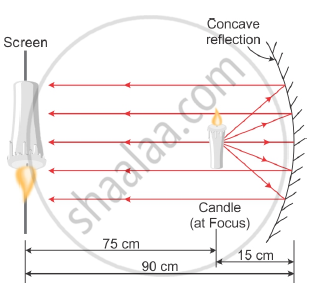# A student wants to project the image of a candle flame on a screen 90 cm in front of a mirror by keeping the flame at a distance of 15 cm from its pole. - Science

A student wants to project the image of a candle flame on a screen 90 cm in front of a mirror by keeping the flame at a distance of 15 cm from its pole.

(a) Suggest the type of mirror he should use.

(b) Determine the linear magnification in this case.

(c) Find the distance between the object and its image.

(d) Draw ray diagram to show the image formation in this case

#### Solution

(a) Concave mirror

(b) Linear magnification of a concave mirror is given by :-

m="-v"/"u"

=(-(-90))/((-15))

= -6

(c) The distance between the object and image = 90 – 15 = 75 cm.

(d) Ray diagram:-Concept: Linear Magnification (M) Due to Spherical Mirrors
Is there an error in this question or solution?